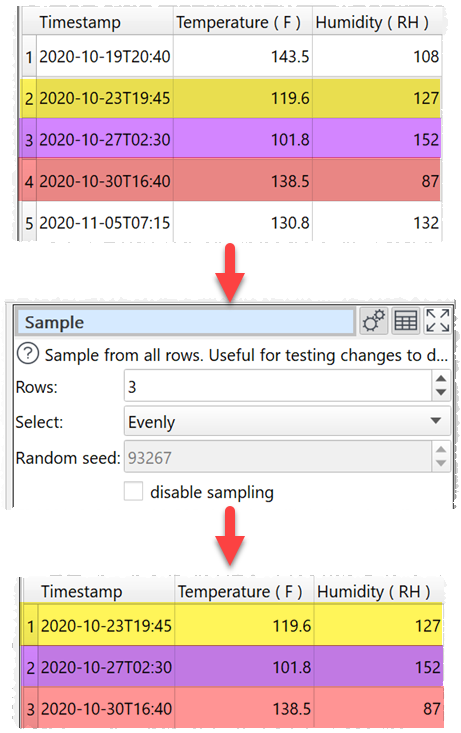﻿ Reference > Transforms > Sample

# Sample

Navigation:  Reference > Transforms >

# Sample

## Description

Selects a subset of rows from the input.

## Example

Sample 3 rows from 5:One.

## Options

Set Rows to the number of rows you want to output. If it is the same or greater than the number of rows in the input, then the input will be unaffected.

Set Select depending on how you want the rows sampled.

Check disable sampling to turn off sampling. If sampling is disabled, the transform does nothing.

## Notes

If you set Select to Randomly (for quality) or Randomly (for speed), rows will be selected using a pseudo-random number generator, initialized with Random seed. Changing the seed will change the rows sampled.

With the same Random seed and the same Select value you should get the same random sampling, even across Windows and Mac versions of Easy Data Transform.

Random (for speed) is significantly faster than Randomly (for quality), but the results may be less random.

If you are transforming a large dataset, then you can use Sample to test a small subset.

If you need to do something more complex than Sample can handle (e.g. keep only rows 500 to 1000) then use Slice or Row Num followed by a Filter. For the most complex cases use Row Num, followed by Javascript, followed by a Filter. E.g. this Javascript function returns 1 for every 10th row between 1000 and 2000 and 0 otherwise:

return \$(Row Num) >= 1000 & \$(Row Num) <= 2000 & \$(Row Num) % 10 == 0;